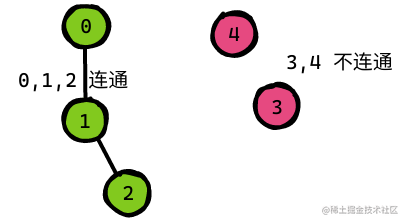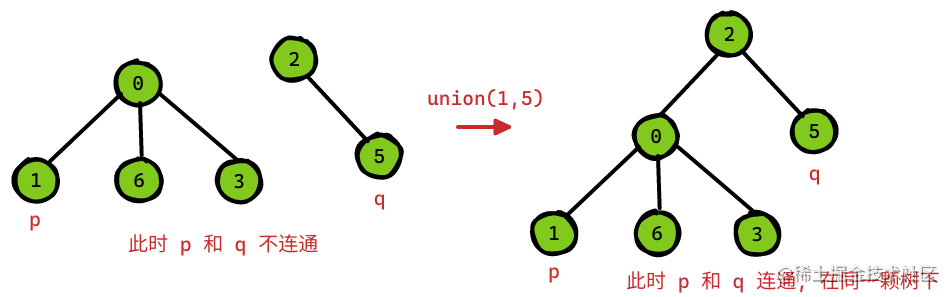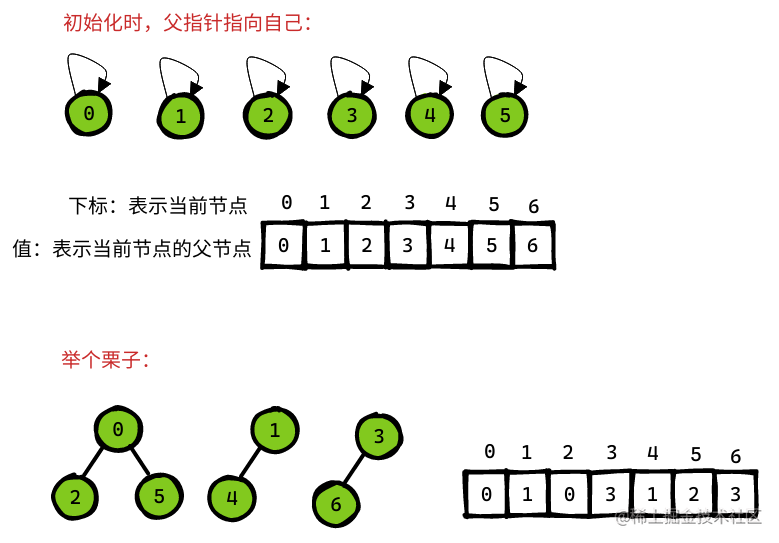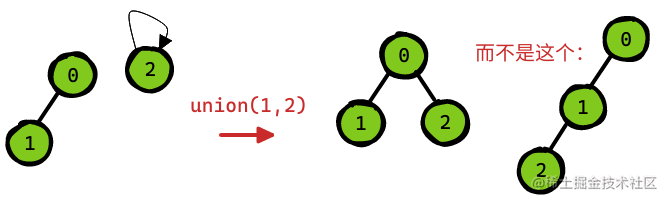# 【算法】并查集总结

## 一、前言1. 自反性：节点 `p``p` 是连通的。
2. 对称性：如果节点 `p``q` 连通，那么 `q``p` 也连通。
3. 传递性：如果节点 `p``q` 连通，`q``r` 连通，那么 `p``r` 也连通。

1. `union(p, q)``p``q` 两节点连通起来： 只需将它们的根节点连通起来即可。
2. `connect(p, q)`判断 `p``q` 两节点是否连通： 判断是否有相同的根节点。1. `union(p, q)`时，小树接到大树下： 需要额外数组 `size[]` 来记录每棵树的节点数量。
2. 进行路径压缩： 尽量挂在根节点下。（每次查找时，进行压缩）``````public class UnionFind {
private int[] parent, size;

public UnionFind(int n) {
parent = new int[n];  // 存储每个节点的父节点
size = new int[n];    // 记录每棵树的 “重量”
for (int i = 0; i < n; ++i) {
parent[i] = i;
size[i] = 1;
}
}

// 1. 查找
public int find(int x) {
return findPathCompressionIterative(x);
}

// 迭代查找，伴随路径压缩
private int findPathCompressionIterative(int x) {
int root = x;
// 1. 查找根节点
while (parent[root] != root) root = parent[root];
// 2. 路径压缩：把查找路径上所有节点的父节点都更新为 根节点root
while (parent[x] != root) {
int tmp = parent[x];
parent[x] = root;
x = tmp;
}
return root;
}

// 2. 联合
public void union(int x, int y) {
int xRoot = find(x);
int yRoot = find(y);
if (xRoot == yRoot) return;

// 小树接到大树下面，较平衡
if (size[xRoot] < size[yRoot]) {
parent[xRoot] = yRoot;
size[yRoot] += size[xRoot];
} else {
parent[yRoot] = xRoot;
size[xRoot] += size[yRoot];
}
}
}

1. `parent[]` 记录每个节点的父节点： 相当于指向父节点的指针，所以 `parent[]` 内实际存储着一个森林
2. `size[]` 数组记录每棵树的重量： 目的是调用 `union` 后树依然拥有平衡性，而不会退化成链表，影响操作效率。
3. `find` 函数中进行路径压缩： 保证任意树的高度保持在常数，使得 `union``connected` `API` 时间复杂度为 `O(1)`

## 二、题目

### （2）省份数量（中）

LeetCode 547

#### 题干分析

``````# 比如说，给你 3 个城市：
0, 1, 2

# 它们对应的连接矩阵 a 是：
1, 0, 0
0, 1, 1
0, 1, 1

# 根据这个矩阵，我们可以知道城市 0 不与城市 1 或城市 2 相连，它自成一个省份。城市 1 与城市 2 相互连接，构成一个省份。

# 因此，这 3 个城市构成了 2 个省份。

#### 思路解法

1. 初始化并查集
2. 构建并查集
1. 查看 `i``j` 是否相连
2. 相连就合并

`AC` 代码：

``````// 方法一： 并查集
// Time: O(n^2), Space: O(n), Faster: 86.14%
public int findCircleNum(int[][] isConnected) {
if (isConnected == null || isConnected.length == 0 ||
isConnected == null || isConnected.length == 0) {
return 0;
}

// 1. 初始化并查集
int n = isConnected.length;
UnionFind uf = new UnionFind(n);

// 2. 构建并查集
for (int i = 0; i < n; ++i) {
// 2.1 查看 i 和 j 是否相连
for (int j = i + 1; j < n; ++j) {
// 2.2 相连就合并
if (isConnected[i][j] == 1) uf.union(i, j);
}
}

return uf.count();
}
public class UnionFind {

private int[] parent, size;
private int cnt; // 统计省数量

public UnionFind(int n) {
parent = new int[n];
size = new int[n];
cnt = n;
for (int i = 0; i < n; ++i) {
parent[i] = i;
size[i] = 1;
}
}

public int find(int x) {
if (parent[x] == x) return x;
parent[x] = find(parent[x]);
return parent[x];
}

// Time: O(a(n)), Space: O(1)
public void union(int x, int y) {
int xRoot = find(x);
int yRoot = find(y);
if (xRoot == yRoot) return;
if (size[xRoot] < size[yRoot]) {
parent[xRoot] = yRoot;
size[yRoot] += size[xRoot];
} else {
parent[yRoot] = xRoot;
size[xRoot] += size[yRoot];
}
--cnt; // 每合并一次，说明能省的 -1
}

public int count() {
// 或者看 parent[x] == x 有几个
return cnt;
}
}

`AC` 代码：

``````// 方法二： 暴力法 DFS
// Time: O(n^2), Space: O(n), Faster: 86.14%
public int findCircleNumDFS(int[][] isConnected) {
if (isConnected == null || isConnected.length == 0 ||
isConnected == null || isConnected.length == 0) {
return 0;
}

int n = isConnected.length;
boolean[] isVisited = new boolean[n];

int cnt = 0;
for (int i = 0; i < n; ++i) {
if (!isVisited[i]) {
++ cnt;
dfs(n, i, isConnected, isVisited);
}
}

return cnt;
}

private void dfs(int n, int i, int[][] isConnected, boolean[] isVisited) {
if (i >= n || i < 0) return;

for (int j = 0; j < n; ++j) {
if (j == i) continue;
if (isConnected[i][j] == 1 && !isVisited[j]) {
isVisited[j] = true;
dfs(n, j, isConnected, isVisited);
}
}
}